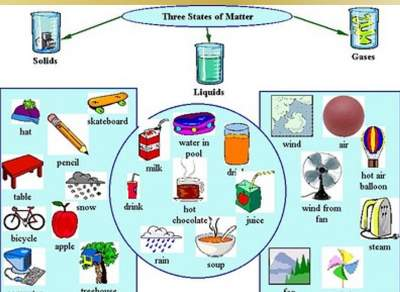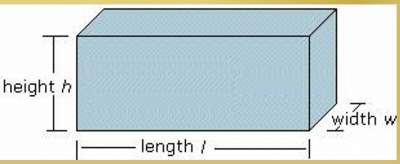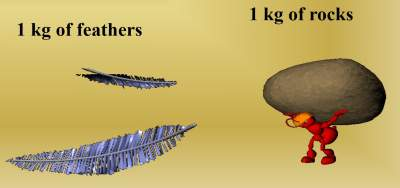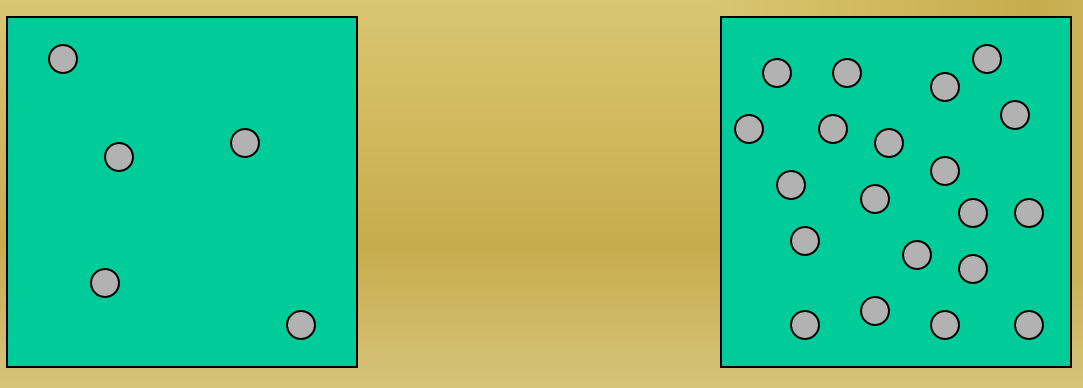# Density

## Mass

Measurement of the amount of matter(or stuff) in an object Measured in grams (g).
There are 3 states of matter:
• Solid
• Liquid
• Gas
See the below illustration for more examples:## Volume

Measurement of the amount of space an object takes up and it is measured for example in milliliters (ml) or cm3### Exercise

Question: See the below illustation and determine which one would have the greater volume? The greater mass?## Density

Density is defined as mass per unit volume.
Question:See the below illustation and determine which square is more dense.#### How to find the density

• Find the mass of the object
• Find the volume of the object
• Density = Mass (g) / Volume (c3)

### Exercise

Assume the mass of an object is 45 grams and it takes up 9 c3 of space, calculate the density. Solution: Density D = M / V = = 45 g / 9 c3 = 5 g/c3

### Ways to Affect Density

Logically speaking:
• Increase the mass → increase density
• Decrease the mass → decrease in density
Practice: Here is an exercise about density